Help protect the Great Barrier Reef with TensorFlow on Kaggle

# Color Space Conversions

## Overview

In computer vision, the selected color space could have a significant the performance of the model. While `RGB` is the most common color space, in manay situations the model performs better when switching to alternative color spaces such as `YUV`, `YCbCr`, `XYZ (CIE)`, etc.

The `tensorflow-io` package provides a list of color space conversions APIs that can be used to prepare and augment the image data.

## Setup

### Install required Packages, and restart runtime

````pip install -q tensorflow-io`
```

The image example used in this tutorial is a cat in the snow, though it could be replaced by any JPEG images.

The following will download the image and save to local disk as `sample.jpg`:

````curl -o sample.jpg -L https://storage.googleapis.com/download.tensorflow.org/example_images/320px-Felis_catus-cat_on_snow.jpg`

`ls -ls sample.jpg`
```
```% Total    % Received % Xferd  Average Speed   Time    Time     Time  Current
100 17858  100 17858    0     0   235k      0 --:--:-- --:--:-- --:--:--  235k
20 -rw-rw-r-- 1 kbuilder kokoro 17858 Oct 27 16:33 sample.jpg
```

## Usage

Read and decode the image into a `uint8` Tensor of shape `(213, 320, 3)`

``````import tensorflow as tf
import tensorflow_io as tfio

print(image.shape, image.dtype)
``````
```(213, 320, 3) <dtype: 'uint8'>
```

The image can be displayed by:

``````import matplotlib.pyplot as plt

plt.figure()
plt.imshow(image)
plt.axis('off')
plt.show()
``````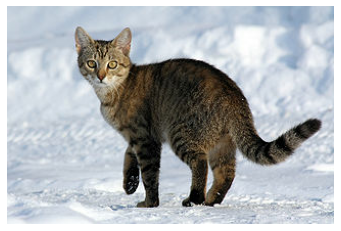### Convert RGB to Grayscale

An `RGB` image can be converted to `Grayscale` to reduce the channel from 3 to 1 with `tfio.experimental.color.rgb_to_grayscale`:

``````grayscale = tfio.experimental.color.rgb_to_grayscale(image)

print(grayscale.shape, grayscale.dtype)

# use tf.squeeze to remove last channel for plt.imshow to display:
plt.figure()
plt.imshow(tf.squeeze(grayscale, axis=-1), cmap='gray')
plt.axis('off')
plt.show()
``````
```(213, 320, 1) <dtype: 'uint8'>
```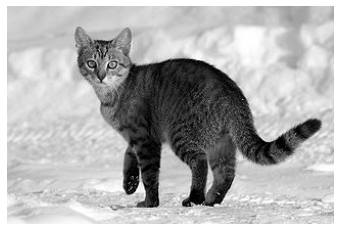### Convert RGB to BGR

Some image software and camera manufacturors might prefer `BGR`, which can be obtained through `tfio.experimental.color.rgb_to_bgr`:

``````bgr = tfio.experimental.color.rgb_to_bgr(image)

print(bgr.shape, bgr.dtype)

plt.figure()
plt.imshow(bgr)
plt.axis('off')
plt.show()
``````
```(213, 320, 3) <dtype: 'uint8'>
```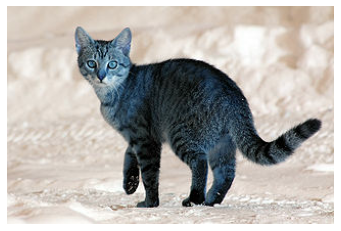### Convert RGB to CIE XYZ

`CIE XYZ` (or `CIE 1931 XYZ` is a common color space used in many image processing programs. The following is the conversion from RGB to `CIE XYZ` through `tfio.experimental.color.rgb_to_xyz`. Note `tfio.experimental.color.rgb_to_xyz` assumes floating point input in the range of `[0, 1]` so additional pre-processing is needed:

``````# convert to float32
image_float32 = tf.cast(image, tf.float32) / 255.0

xyz_float32 = tfio.experimental.color.rgb_to_xyz(image_float32)

# convert back uint8
xyz = tf.cast(xyz_float32 * 255.0, tf.uint8)

print(xyz.shape, xyz.dtype)

plt.figure()
plt.imshow(xyz)
plt.axis('off')
plt.show()
``````
```(213, 320, 3) <dtype: 'uint8'>
```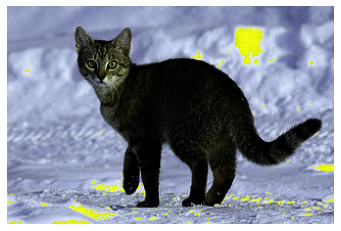### Convert RGB to YCbCr

Finally, `YCbCr` is the default color space in many video systems. Converting to `YCbCr` could be done through `tfio.experimental.color.rgb_to_ycbcr`:

``````ycbcr = tfio.experimental.color.rgb_to_ycbcr(image)

print(ycbcr.shape, ycbcr.dtype)

plt.figure()
plt.imshow(ycbcr, cmap='gray')
plt.axis('off')
plt.show()
``````
```(213, 320, 3) <dtype: 'uint8'>
```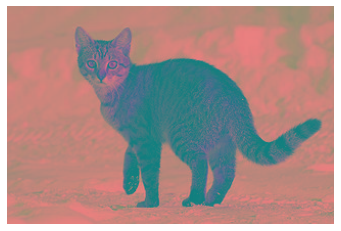What is more interesting, though, is that `YCbCr` could be decomposed into `Y'` (luma), `Cb` (blue-difference chroma), and `Cr` (red-difference chroma) components with each component carry perceptually meaningful information:

``````y, cb, cr = ycbcr[:,:,0], ycbcr[:,:,1], ycbcr[:,:,2]

# Y' component
plt.figure()
plt.imshow(y, cmap='gray')
plt.axis('off')
plt.show()

# Cb component
plt.figure()
plt.imshow(cb, cmap='gray')
plt.axis('off')
plt.show()

# Cr component
plt.figure()
plt.imshow(cr, cmap='gray')
plt.axis('off')
plt.show()
``````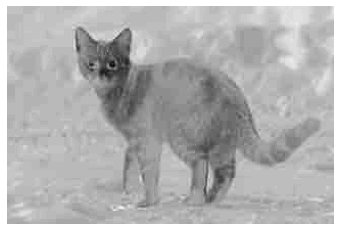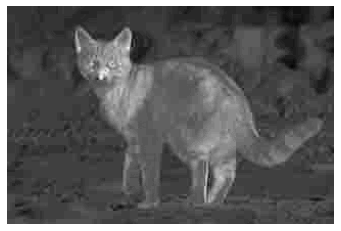[{ "type": "thumb-down", "id": "missingTheInformationINeed", "label":"Missing the information I need" },{ "type": "thumb-down", "id": "tooComplicatedTooManySteps", "label":"Too complicated / too many steps" },{ "type": "thumb-down", "id": "outOfDate", "label":"Out of date" },{ "type": "thumb-down", "id": "samplesCodeIssue", "label":"Samples / code issue" },{ "type": "thumb-down", "id": "otherDown", "label":"Other" }]
[{ "type": "thumb-up", "id": "easyToUnderstand", "label":"Easy to understand" },{ "type": "thumb-up", "id": "solvedMyProblem", "label":"Solved my problem" },{ "type": "thumb-up", "id": "otherUp", "label":"Other" }]